# Conversion Functions in Pandas DataFrame

• Last Updated : 25 Jul, 2019

Python is a great language for doing data analysis, primarily because of the fantastic ecosystem of data-centric python packages. Pandas is one of those packages and makes importing and analyzing data much easier. In this article, we are using “`nba.csv`” file to download the CSV, click here.### Cast a pandas object to a specified dtype

DataFrame.astype() function is used to cast a pandas object to a specified dtype. `astype()` function also provides the capability to convert any suitable existing column to categorical type.

Code #1: Convert the Weight column data type.

 `# importing pandas as pd``import` `pandas as pd`` ` `# Making data frame from the csv file``df ``=` `pd.read_csv(``"nba.csv"``)`` ` `# Printing the first 10 rows of ``# the data frame for visualization`` ` `df[:``10``]`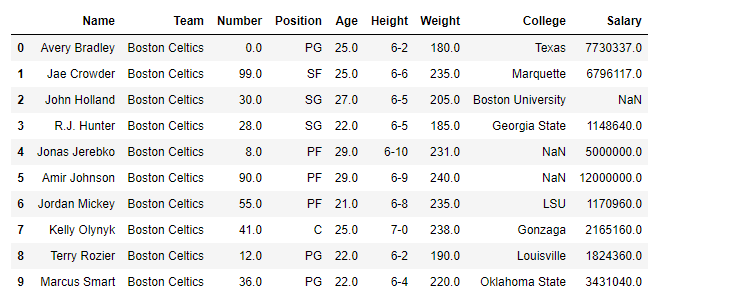As the data have some “nan” values so, to avoid any error we will drop all the rows containing any `nan `values.

 `# drop all those rows which ``# have any 'nan' value in it.``df.dropna(inplace ``=` `True``)`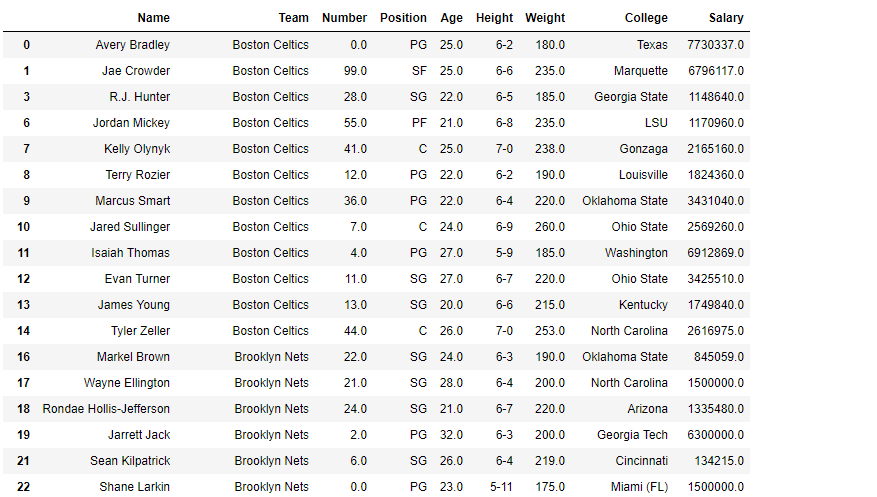`# let's find out the data type of Weight column``before ``=` `type``(df.Weight[``0``])`` ` `# Now we will convert it into 'int64' type.``df.Weight ``=` `df.Weight.astype(``'int64'``)`` ` `# let's find out the data type after casting``after ``=` `type``(df.Weight[``0``])`` ` `# print the value of before``before`` ` `# print the value of after``after`

Output: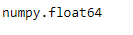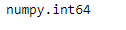`# print the data frame and see``# what it looks like after the change``df`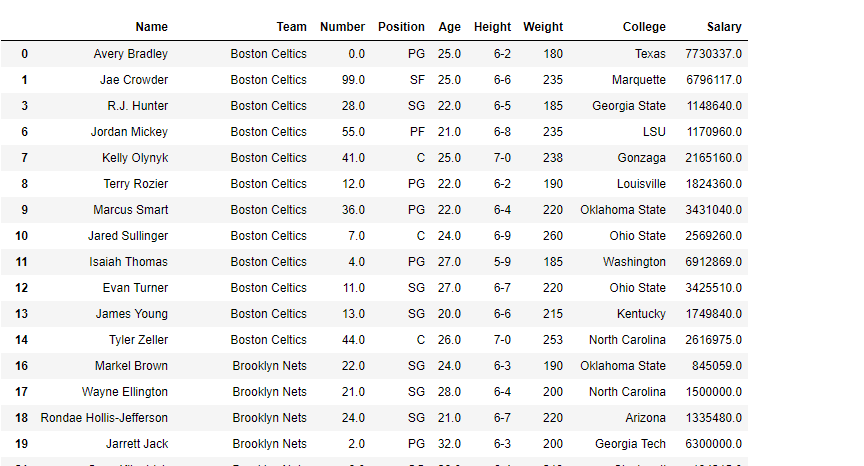### Infer better data type for input object column

DataFrame.infer_objects() function attempts to infer better data type for input object column. This function attempts soft conversion of object-dtyped columns, leaving non-object and unconvertible columns unchanged. The inference rules are the same as during normal Series/DataFrame construction.

Code #1: Use `infer_objects()` function to infer better data type.

 `# importing pandas as pd``import` `pandas as pd`` ` `# Creating the dataframe ``df ``=` `pd.DataFrame({``"A"``:[``"sofia"``, ``5``, ``8``, ``11``, ``100``],``                   ``"B"``:[``2``, ``8``, ``77``, ``4``, ``11``],``                   ``"C"``:[``"amy"``, ``11``, ``4``, ``6``, ``9``]})`` ` `# Print the dataframe``print``(df)`

Output :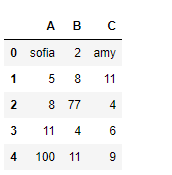Let’s see the dtype (data type) of each column in the dataframe.

 `# to print the basic info``df.info()`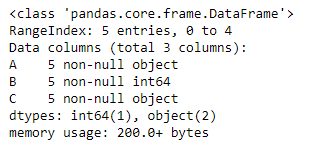As we can see in the output, first and third column is of `object` type. whereas the second column is of `int64` type. Now slice the dataframe and create a new dataframe from it.

 `# slice from the 1st row till end``df_new ``=` `df[``1``:]`` ` `# Let's print the new data frame``df_new`` ` `# Now let's print the data type of the columns``df_new.info()`

Output :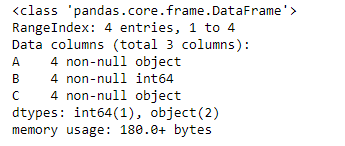As we can see in the output, column “A” and “C” are of object type even though they contain integer value. So, let’s try the `infer_objects()` function.

 `# applying infer_objects() function.``df_new ``=` `df_new.infer_objects()`` ` `# Print the dtype after applying the function``df_new.info()`

Output :Now, if we look at the dtype of each column, we can see that the column “A” and “C” are now of `int64` type.

### Detect missing values

DataFrame.isna() function is used to detect missing values. It return a boolean same-sized object indicating if the values are NA. NA values, such as None or numpy.NaN, gets mapped to True values. Everything else gets mapped to False values. Characters such as empty strings ” or numpy.inf are not considered NA values (unless you set pandas.options.mode.use_inf_as_na = True).

Code #1: Use `isna()` function to detect the missing values in a dataframe.

 `# importing pandas as pd``import` `pandas as pd`` ` `# Creating the dataframe ``df ``=` `pd.read_csv(``"nba.csv"``)`` ` `# Print the dataframe``df`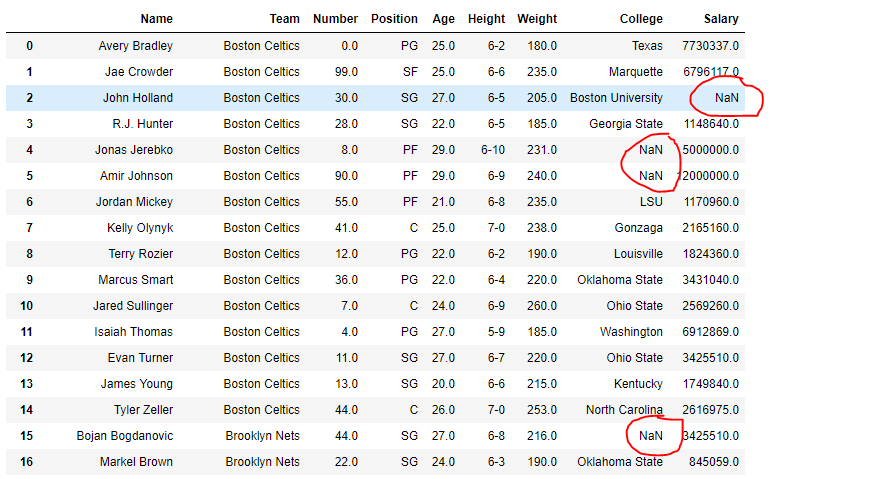Lets use the `isna()` function to detect the missing values.

 `# detect the missing values``df.isna()`

Output :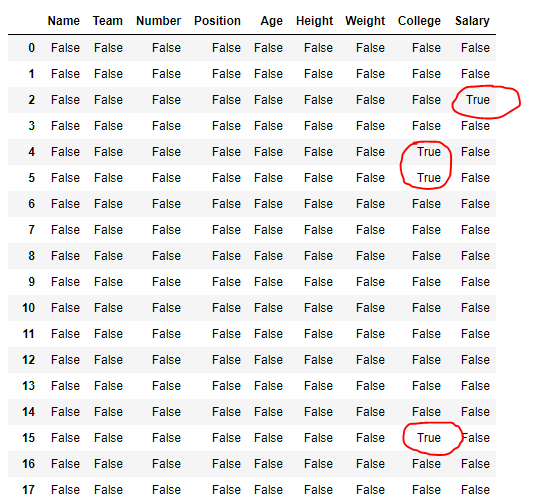In the output, cells corresponding to the missing values contains true value else false.

### Detecting existing/non-missing values

DataFrame.notna() function detects existing/ non-missing values in the dataframe. The function returns a boolean object having the same size as that of the object on which it is applied, indicating whether each individual value is a na value or not. All of the non-missing values gets mapped to true and missing values get mapped to false.

Code #1: Use `notna()` function to find all the non-missing value in the dataframe.

 `# importing pandas as pd``import` `pandas as pd`` ` `# Creating the first dataframe ``df ``=` `pd.DataFrame({``"A"``:[``14``, ``4``, ``5``, ``4``, ``1``],``                   ``"B"``:[``5``, ``2``, ``54``, ``3``, ``2``], ``                   ``"C"``:[``20``, ``20``, ``7``, ``3``, ``8``],``                   ``"D"``:[``14``, ``3``, ``6``, ``2``, ``6``]})`` ` `# Print the dataframe``print``(df)`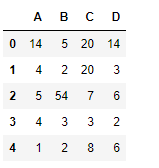Let’s use the `dataframe.notna()` function to find all the non-missing values in the dataframe.

 `# find non-na values``df.notna()`

Output :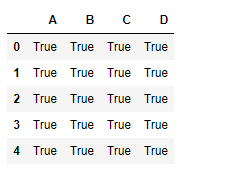As we can see in the output, all the non-missing values in the dataframe has been mapped to true. There is no false value as there is no missing value in the dataframe.

#### Methods for conversion in DataFrame

FunctionDescription
DataFrame.convert_objects()Attempt to infer better dtype for object columns.
DataFrame.copy()Return a copy of this object’s indices and data.
DataFrame.bool()Return the bool of a single element PandasObject.

My Personal Notes arrow_drop_up